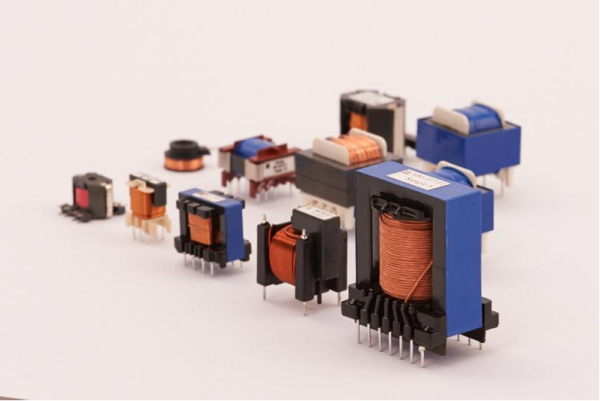# Calculated power of the Transformer

##### author: MagTop
17/06/2022Transformer power = output voltage X output current
Single-phase transformer power is obtained by the total power consumption *120% (efficiency calculated by 80%).
Three-phase transformer power calculation is as follows (take phase voltage 220V, line voltage 380V as an example) :
1, three-phase rated power =1.732* rated current * rated line voltage (380V) =3* rated current * rated phase voltage (220V).
2, three-phase power is different, according to the maximum power multiplied by 3 calculation, such as, A phase 9KW, B phase 10KW, C phase 11KW, P=3*11=33KW.
3, transformer power factor is generally 0.8 (there are also 0.7), then, in the above example, the total power of the transformer =33/0.8= 41.25kW.